### How To Use The Integral Function In Excel

The integration is part of the important concepts that associate with mathematic, and is part of the main operations in calculus. It has to be given the function f of a variable x that is real, as well as the interval which is a, b, of that real line, and this is the definite integral:This has been informally defined as signed area of region that is in x/y-plane, and its boundary by graph of f, x-axis, as well as vertical lines x that is a, and the x that is equal to b. The term known as integral could also refer to related notion of antiderivative, which is a function F, and whose derivative is the function of that is given. In such case, an indefinite integral is defined, and also written like this:You are going to work with integral of a simple calculation following this simple steps below.

### Data LayoutYou should decide the dx value, which is 0,1. This is showing in the picture above.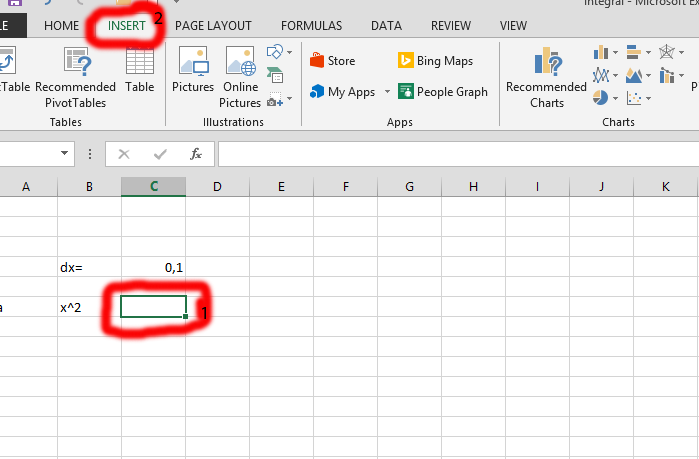Click on the column that is beside the x^2, and labeled as number

1. Click on Insert tab, the one labeled as number 2.

Click on the equation, which is marked in red. Do not click on the arrow, just on the equation itself.Click on the integral (labeled number 1) showing once you have clicked on the equation in previous step, and then click on the kind of integral you would like, in this case, the one labeled number 2 is chosen.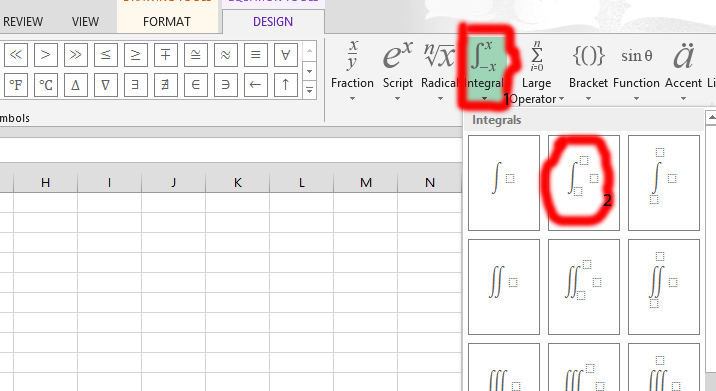Place the value that are relevant to the integral function. As you could see in the one marked in red, and labeled as number 1 is going to have a detail of your choice. On top of the f there is a, while on the end, there is a 0, and in the middle there is an x. You should click on the line (where number 2 is marked), and expand the whole row to fit the integral equation that has been inserted.

Tip: you should type in the x2 (marked in the number 1), using an insert symbol (which is in the insert tab).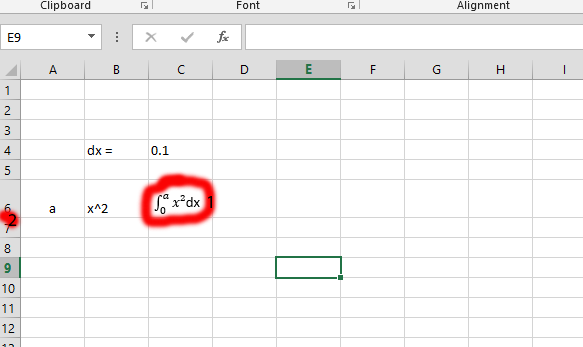### Integral Calculation

Add 0 (in this case in a7) with the 0,1 – the dx.

Information: If you see any error calculating, you should change from 0,1 to 0.1 to continue.Click on the small square beside the number 1, using your mouse. Drag it down to the end, as you wish. In this case, it is dragged to a20.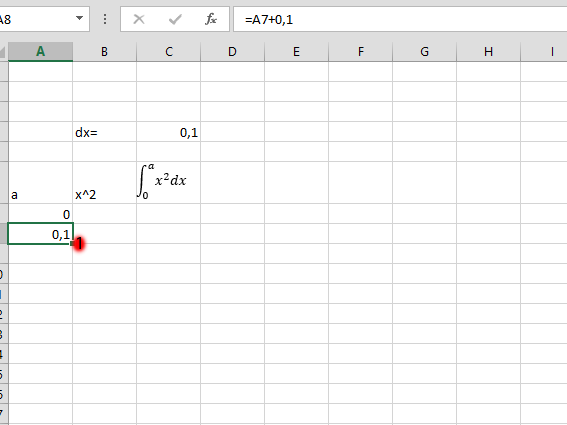Click on the column in the row of the ^2, and add the content in “a” row to multiply with ^2. Repeat the step 2 – 2 with the A row, as it is in this case. Check the picture for clarification.Type in the columns that are relevant to the integral you are calculating. You would see the marked columns, as well as some added number.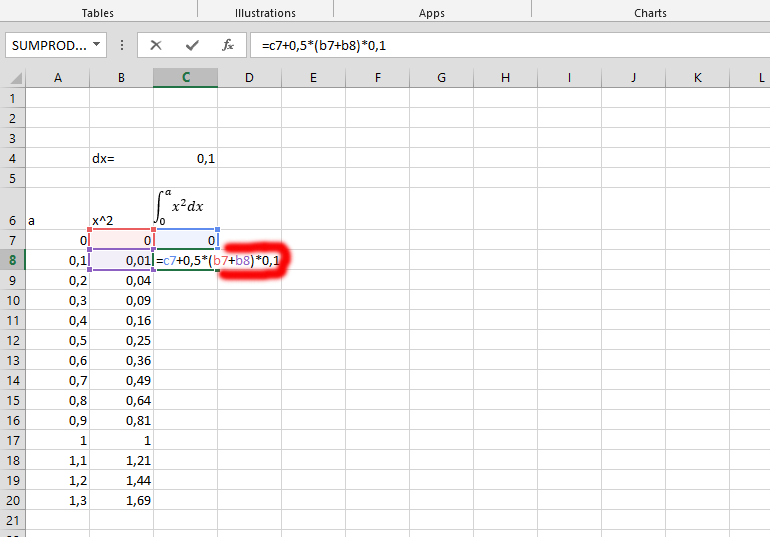Click on the integral you have just calculated, and now click on the small square that is in the marked area and on the left side of the one labeled number 1, and drag it down to save yourself the stress of calculating one after the other.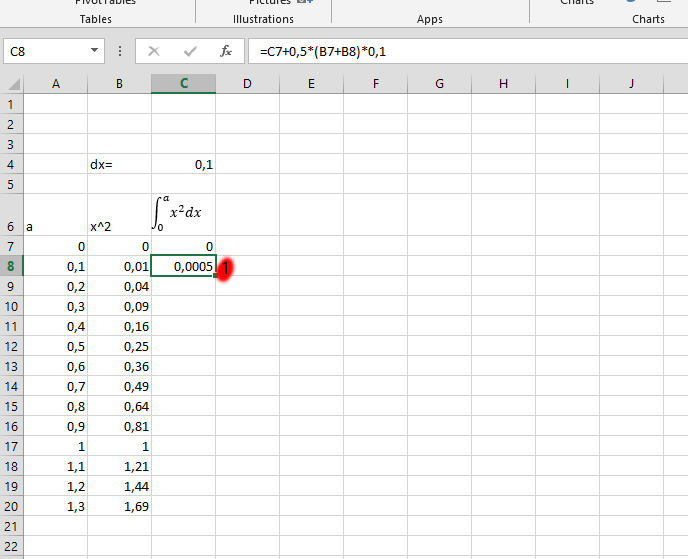### Design (Optional)

Mark the rows you would like to display in a specific design.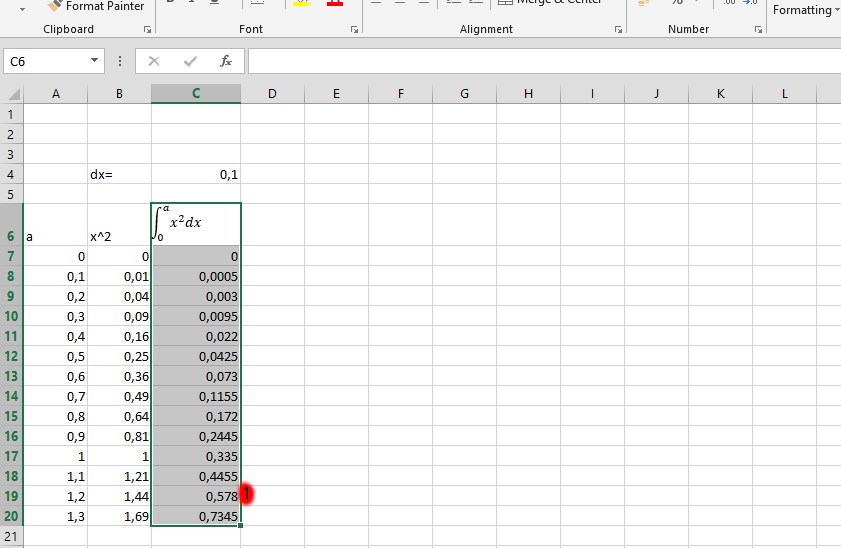Click on the INSERT tab, the one labeled as number 1, and then choose the type of chart you would like to display, which is labeled as number 2.Choose the design you would prefer for your integral chart.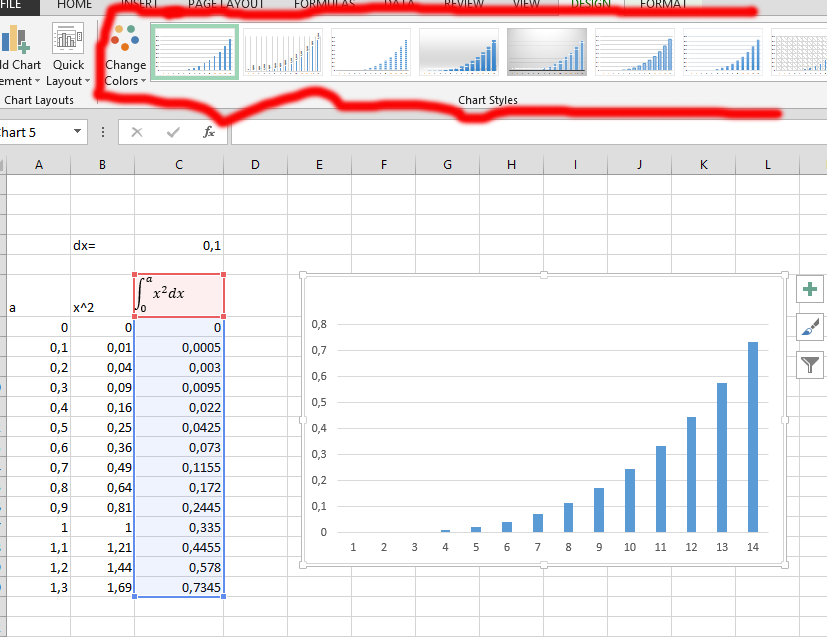Insert column chart of your integral function and write the title you would like to give the chart.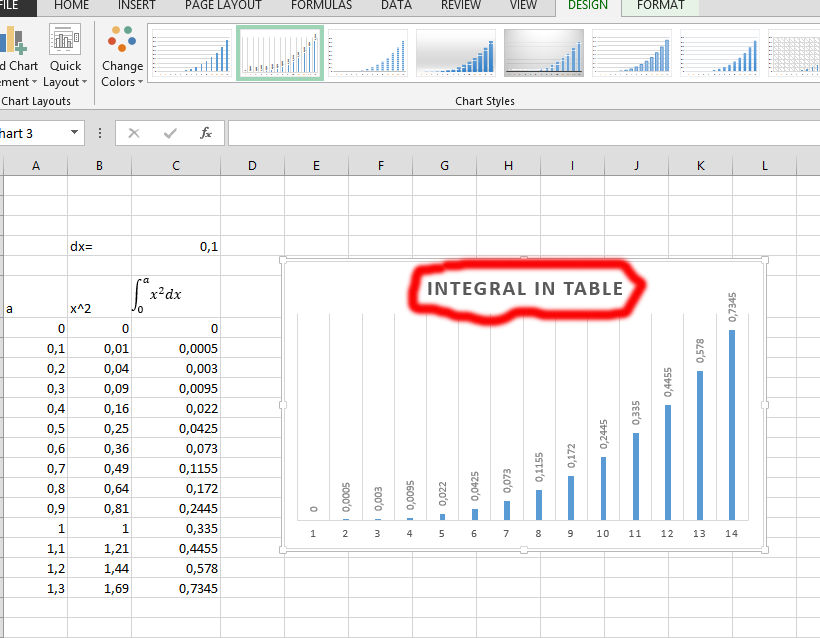```Further reading: